# Python+OpenCV 检测灯光亮点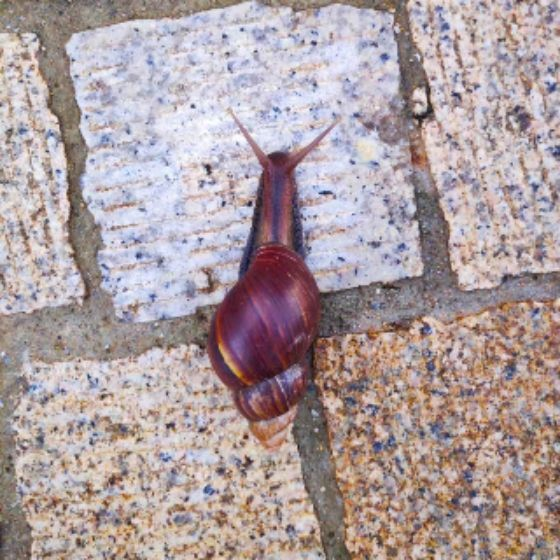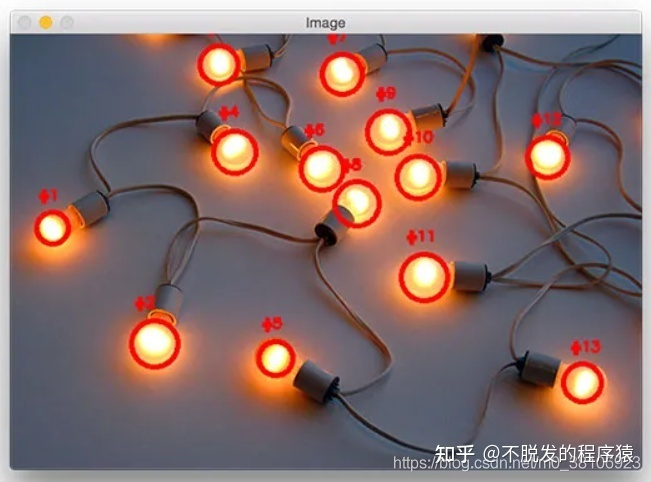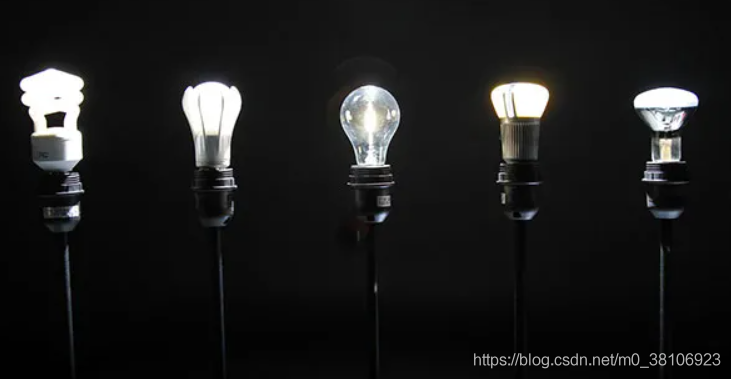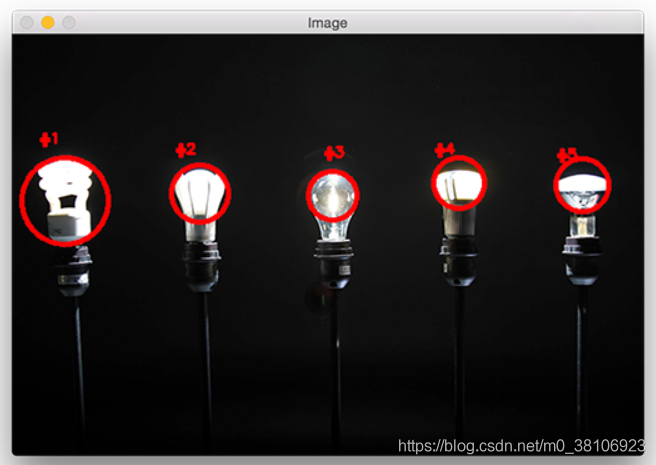# import the necessary packagesfrom imutils import contoursfrom skimage import measureimport numpy as npimport argparseimport imutilsimport cv2# construct the argument parse and parse the argumentsap = argparse.ArgumentParser()ap.add_argument("-i", "--image", required=True,  help="path to the image file")args = vars(ap.parse_args())

#load the image, convert it to grayscale, and blur itimage = cv2.imread(args["image"])gray = cv2.cvtColor(image, cv2.COLOR_BGR2GRAY)blurred = cv2.GaussianBlur(gray, (11, 11), 0)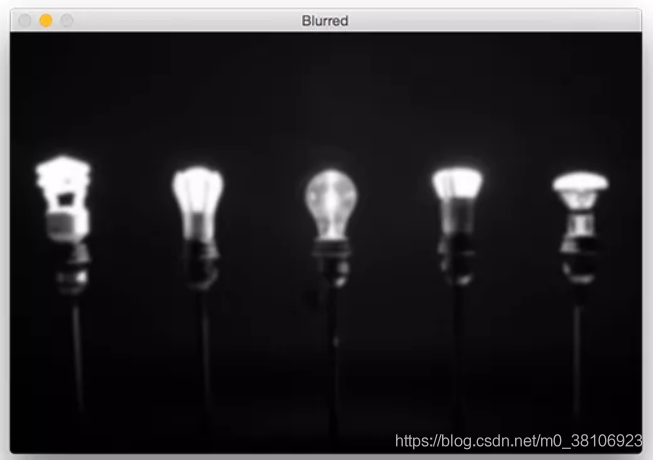# threshold the image to reveal light regions in the# blurred imagethresh = cv2.threshold(blurred, 200, 255, cv2.THRESH_BINARY)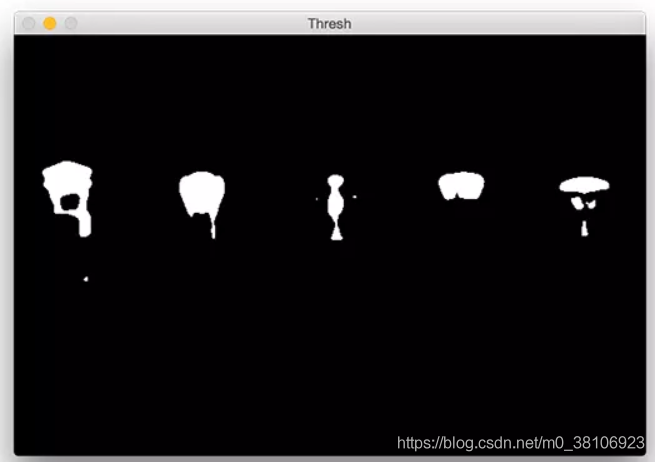# perform a series of erosions and dilations to remove# any small blobs of noise from the thresholded imagethresh = cv2.erode(thresh, None, iterations=2)thresh = cv2.dilate(thresh, None, iterations=4)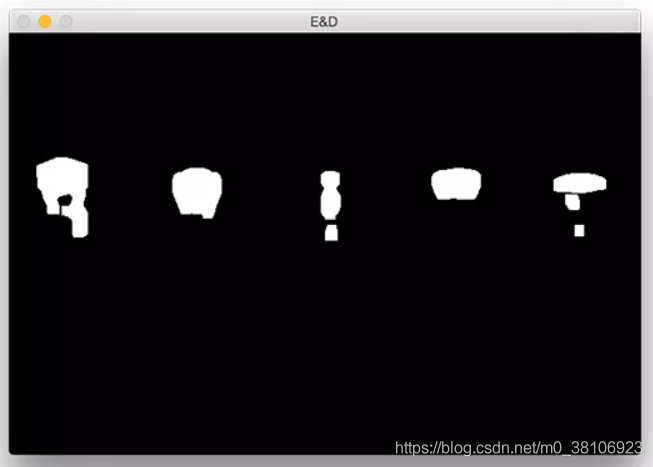# perform a connected component analysis on the thresholded# image, then initialize a mask to store only the "large"# componentslabels = measure.label(thresh, neighbors=8, background=0)mask = np.zeros(thresh.shape, dtype="uint8")# loop over the unique componentsfor label in np.unique(labels):  # if this is the background label, ignore it  if label == 0:    continue  # otherwise, construct the label mask and count the  # number of pixels   labelMask = np.zeros(thresh.shape, dtype="uint8")  labelMask[labels == label] = 255  numPixels = cv2.countNonZero(labelMask)  # if the number of pixels in the component is sufficiently  # large, then add it to our mask of "large blobs"  if numPixels > 300:    mask = cv2.add(mask, labelMask)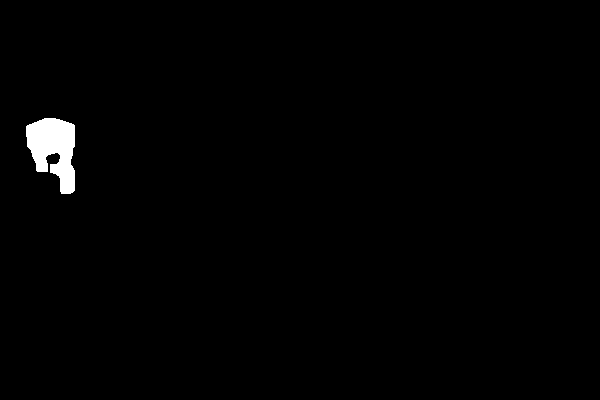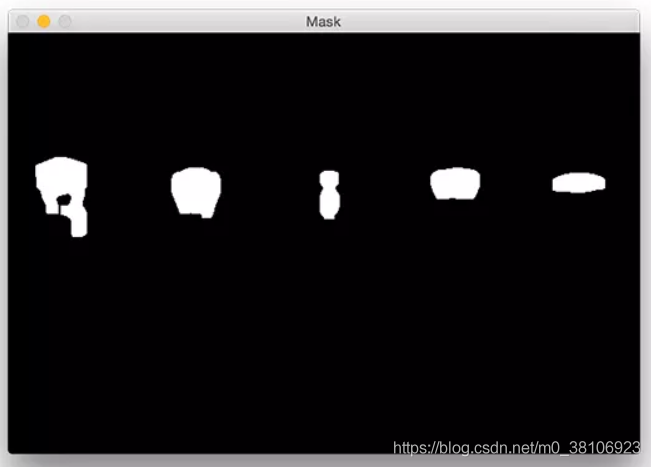# find the contours in the mask, then sort them from left to# rightcnts = cv2.findContours(mask.copy(), cv2.RETR_EXTERNAL,  cv2.CHAIN_APPROX_SIMPLE)cnts = imutils.grab_contours(cnts)cnts = contours.sort_contours(cnts)# loop over the contoursfor (i, c) in enumerate(cnts):  # draw the bright spot on the image  (x, y, w, h) = cv2.boundingRect(c)  ((cX, cY), radius) = cv2.minEnclosingCircle(c)  cv2.circle(image, (int(cX), int(cY)), int(radius),    (0, 0, 255), 3)  cv2.putText(image, "#{}".format(i + 1), (x, y - 15),    cv2.FONT_HERSHEY_SIMPLEX, 0.45, (0, 0, 255), 2)# show the output imagecv2.imshow("Image", image)cv2.waitKey(0)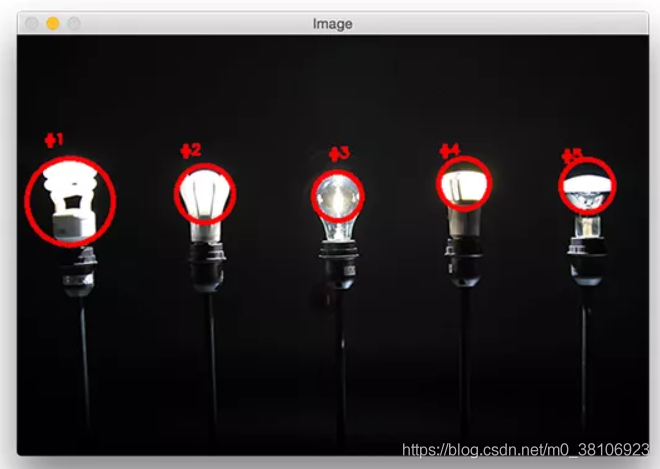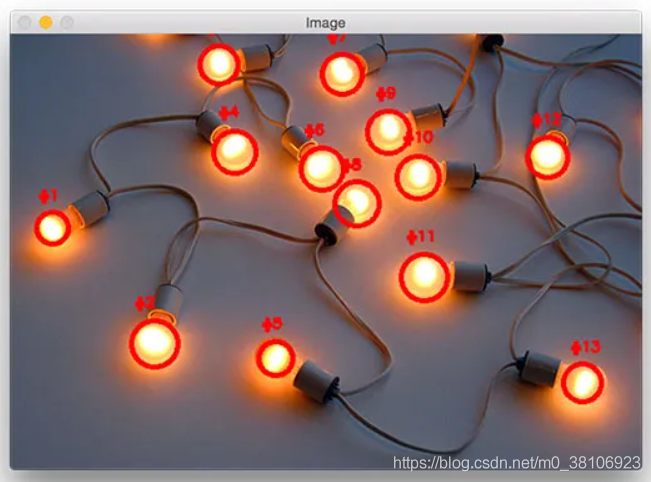### 不脱发的程序猿

【研究方向】物联网、嵌入式、AI、Python 2018.02.09 加入

【公众号】美男子玩编程

## 评论